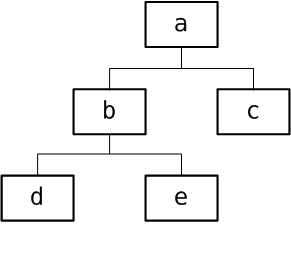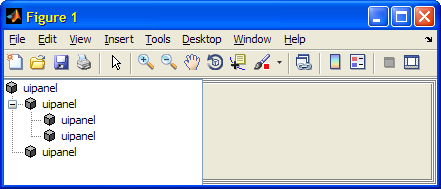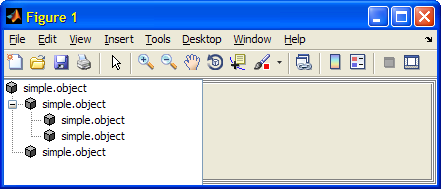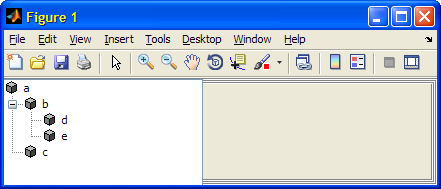# Hierarchical Systems with UDD

Once again I welcome guest blogger Donn Shull, who continues his multi-part series about Matlab’s undocumented UDD objects.

We have looked at the tools for working with UDD classes, and created a simple UDD class. Today I shall show how to create a hierarchy of UDD objects.

### Creating hierarchical structures with UDD objects

UDD is the foundation for both Handle Graphics (HG) and Simulink. Both are hierarchical systems. It stands to reason that UDD would offer support for hierarchical structures. It is straightforward to connect UDD objects together into searchable tree structures. All that is necessary is a collection of UDD objects that don’t have any methods or properties named `'connect', 'disconnect', 'up', 'down', 'left', 'right'` or `'find'`.

We illustrate the technique by creating a hierarchy of `simple.object`s as shown in the following diagram:Sample UDD objects hierarchy

To begin we create five instances of the `simple.object` class from the previous article:

```% Remember that simple.object accepts a name and a value a = simple.object('a', 1); b = simple.object('b', 1); c = simple.object('c', 0); d = simple.object('d', 1); e = simple.object('e', 1);```

To form the structure we use the connect method. We can use either dot notation or the Matlab syntax:

```% Dot-notation examples: a.connect(b, 'down'); b.connect(a, 'up'); % alternative to the above   % Matlab notation examples: connect(a, b, 'down'); connect(b, a, 'up'); % alternative to the above```

Next, connect node c into our hierarchy. There are several options here: We can use ‘down’ to connect a to c. Or we could use ‘up’ to connect c to a. Similarly, we can use either ‘left’ or ‘right’ to connect b and c. Here’s one of the many possible ways to create our entire hierarchy:

```b.connect(a, 'up'); c.connect(b, 'left'); d.connect(b, 'up'); e.connect(d, 'left');```

### Inspecting UDD hierarchy structures

We now have our structure and each object knows its connection to other objects. For example, we can inspect b’s connections as follows:

```>> b.up ans = Name: a Value: 1.000000   >> b.right ans = Name: c Value: 0.000000   >> b.down ans = Name: d Value: 1.000000```

We can search our structure by using an undocumented form of the built-in find command. When used with connected UDD structures, find can be used in the following form:

`objectArray = find(startingNode, 'property', 'value', ...)`

To search from the top of our hierarchy for objects of type `simple.object` we would use:

```>> find(a, '-isa', 'simple.object') ans = simple.object: 5-by-1 % a, b, c, d, e```

Which returns all the objects in our structure, since all of them are `simple.object`s. If we repeat that command starting at b we would get:

```>> find(b, '-isa', 'simple.object') ans = simple.object: 3-by-1 % b, d, e```

find searches the structure downward from the current node. Like many Matlab functions, find can be used with multiple property value pairs, so if we want to find `simple.object` objects in our structure with Value property =0, we would use the command:

```>> find(a, '-isa', 'simple.object', 'Value', 0) ans = Name: c Value: 0.000000```

### Visualizing a UDD hierarchy

Hierarchical structures are also known as tree structures. Matlab has an undocumented function for visualizing and working with trees namely uitree. Yair has described uitree in a series of articles. Rather than following the techniques in shown in Yair’s articles, we are going to use a different method that will allow us to introduce the following important techniques for working with UDD objects:

• Subclassing, building your class on the foundation of a parent class
• Using meta-properties GetfFunction and SetFunction

Because the steps shown below will subclass an HG class, they will modify our `simple.object` class and probably make it unsuitable for general use. Yair has shown that uitree is ready made for displaying HG trees and we saw above that HG is a UDD system. We will use the technique from `uitools.uibuttongroup` to make our `simple.object` class a subclass of the HG class `hg.uipanel`. Modify the class definition file as follows:

```% class definition superPackage = findpackage('hg'); superClass = findclass(superPackage, 'uipanel'); simpleClass = schema.class(simplePackage, 'object',superClass);```

Now we can either issue the clear classes command or restart Matlab and then recreate our structure. The first thing that you will notice is that when we create the first `simple.object` that a figure is also created. This is expected and is the reason that this technique is not useful in general. We will however use this figure to display our structure with the following commands:

```t = uitree('v0', 'root', a); drawnow; t.expand(t.getRoot); drawnow; t.expand(t.getRoot.getFirstChild);```Simple structure presented in a Matlab uitree

The label on each of our objects is ‘uipanel’ and this is probably not what we want. If we inspect our object or its `hg.uipanel` super-class (note: this would be a great time to use Yair’s uiinspect utility), we can see there is a Type property that has a value of ‘uipanel’. Unfortunately this property is read-only, so we cannot change it. We can however overload it by placing a schema.prop in our class definition named Type. This will allow us to overload or replace the parent’s Type property with our own definition:

```p = schema.prop(simpleClass, 'Type', 'string'); p.FactoryValue = 'simple.object';```

Once again, issue the clear classes command or restart Matlab, then recreate our structure. Our tree now has each node labeled with the ‘simple.object’ label:Corrected node names for our UDD structure

This is a little more descriptive but what would really be nice is if we could label each node with the value of the Name property. As luck would have it, we can do just that. When we add a property to a UDD class we are adding an object of type `schema.prop`. So our properties have their own properties and methods (so-called meta-data). We are going to set the GetFunction property of our Type property. GetFunction holds a handle of the function to be called whenever the property is accessed:

```p = schema.prop(simpleClass, 'Type', 'string'); p.GetFunction = @getType;```

The prototype for the function that GetFunction references has three inputs and one output: The inputs are the handle of the object possessing the property, the value of that property, and the property object. The output is the value that will be supplied when the property is accessed. So our GetFunction can be written to supply the value of the Name property whenever the Type property value is being read:

```function propVal = getType(self, value, prop) propVal = self.Name; end```

Alternately, as a single one-liner in the schema definition file:

`p.GetFunction = @(self,value,prop) self.Name;`

Similarly, there is a corresponding SetFunction that enables us to intercept changes to a property’s value and possibly disallow invalid values.

With these changes when we recreate our uitree we obtain:### A Java class for UDD trees

We will have more to say about the relationship between UDD and Java in a future article. For now we simply note that the `com.mathworks.jmi.bean.UDDObjectTreeModel` class in the JMI package provides some UDD tree navigation helper functions. Methods include getChild, getChildCount, getIndexOfChild and getPathToRoot. The `UDDObjectTreeModel` constructor requires one argument, an instance of your UDD tree root node:

```% Create a UDD tree-model instance >> uddTreeModel = com.mathworks.jmi.bean.UDDObjectTreeModel(a);   % Get index of child e and its parent b: >> childIndex = uddTreeModel.getIndexOfChild(b, e) childIndex = 1   % Get the root's first child (#0): >> child0 = uddTreeModel.getChild(a, 0) child0 = Name: b Value: 1.000000   % Get the path from node e to the root: >> path2root = uddTreeModel.getPathToRoot(e) path2root = com.mathworks.jmi.bean.UDDObject[]: [simple_objectBeanAdapter2] % <= a [simple_objectBeanAdapter2] % <= b [simple_objectBeanAdapter2] % <= e   >> path2root(3) ans = Name: e Value: 1.000000```

We touched on a few of the things that you can do by modifying the properties of a `schema.prop` in this article. In the following article we will take a more detailed look at this essential class.Print

### 5 Responses to Hierarchical Systems with UDD

1.Sebastian Hölz says:

Hi Donn, thanks for this interesting series of articles. It touches a field that I’m currently investigating, but where I can not find a suitable solution yet.

Simple example:
Suppose I create a UDD-subclass of HG-axes named mAxis and want to be able to restrict the user to certain xlims, e.g. only multiples of 10. How can I intercept a call like

`>> set(H, 'xlim', [11 20]) % Where H is a mAxis object`

On the one hand side, if I make xlim a property of the class (using “schema.prop …” in the schema.m-file) and supply the according SetFunction, I can no longer pass the property to the “superclass”, i.e. the axis object.

On the other hand Matlab will not let me specify a SetFunction in the mAxis.m, because the xlim property is protected.

OK, maybe you can hint me to some solution.

Cheers

Sebastian

•Donn Shull says:

@Sebastian – Normally you can overload a propertyof the superclass in a subclass. I think the problem in this case MATLAB will not allow you to set the type of your overloaded XLim property to axesXLimType and no other type will work. XLim does generate Events so you could try something like:

`lis = handle.listener(a, a.findprop('XLim'), 'PropertyPostSet', 'a.XLim = [11,20];')`

In the upcoming section on UDD events we will show how to placecode like this in a a class.

Best Regards,

Donn

•Sebastian Hölz says:

Your example using ‘PropertyPostSet’ works. I thought that this would lead to a racing condition, but it doesn’t. I will work from here on …

Thanks

Sebastian

PS to anyone, who wants to try something similar:

1) Define property “PL” (-> PropListener) in schema.m file:

p = schema.prop(MyClass, ‘PropListener’, ‘handle vector’);

2) Specify ‘PropertyPostSet’ listener and nested function to handle the property change in the MyClass.m – file.

function obj = MyClass(varargin)

obj = MyPackage.MyClass;
obj.PL=handle.listener(obj, obj.findprop(‘XLim’), ‘PropertyPostSet’,@MF);

function MF(~, in)
obj.xlim = round(in.NewValue);
end

end

– The handle to the listener is saved in property “PL”, otherwise the function “MF” would not be called. This is similar to what is done in the “linkprop” command (s. Matlab documentation).

– Since “MF” is specified as nested function, obj is still in scope and the new value (in.NewValue) can be checked, altered and passed to the object.

2.Matt S says:

Hi,

I am trying to create a simple UDD class similar to this. The class is the same as simple.object with simple.object being a subclass of uipanel. I am trying to include a uicontrol slider on the panel during construction. When I open up Matlab, the first time I run the constructor, I always get the error: The class name ‘ScrollablePanel’ already exists. If I run it again, it works without error. Is there a way I can make it work the first time? If it helps, here is the constructor:

```function this = ScrollablePanel(varargin) this = uiStrider.ScrollablePanel; set(this,varargin{:}); set(this,'SliderHandle',uicontrol('Parent',handle(this), 'Style','Slider','Units','Pixels')); end```

Thanks for doing this website. I use it all the time,
Matt